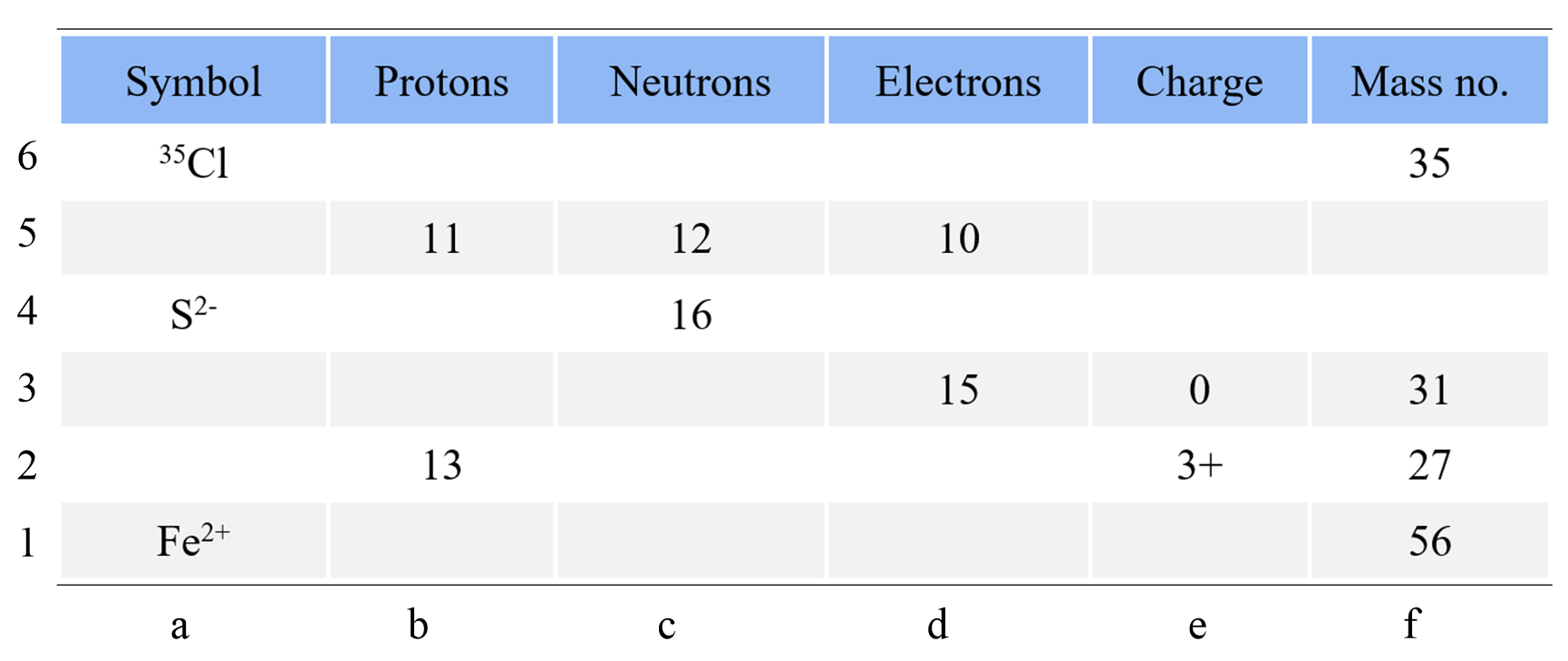## Atoms, Molecules, and Ions

Before we start with some examples of calculating the number of protons, neutrons, and electrons, let’s recall what information we get about a particular element from the periodic table. For example, if we look up potassium, K in the periodic table, we will see the following numbers: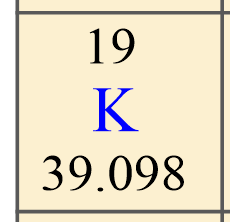The first, you want to identify is the atomic number of the element because this is unique for each element. This is the number usually given on the top of the symbol, and, in this case, it is 19.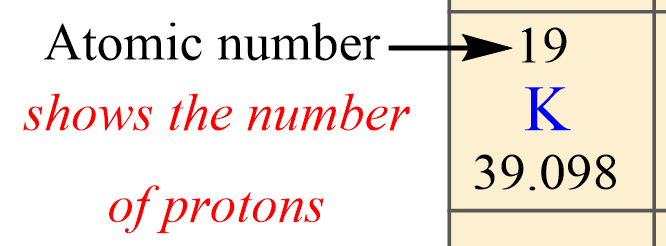So, potassium has 19 protons, and this is true for all its isotopes. There cannot be another element with the atomic number 19.

Next, on the button, we have the average atomic mass of potassium which is equal to 39.098. This is the average atomic mass of all the isotopes of potassium and that is why it is not a whole number.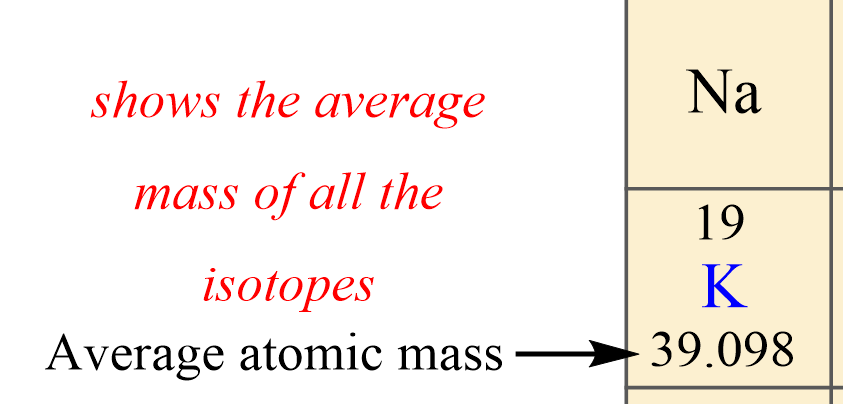If you are given data stating that the atomic mass of potassium is, for example, 39, then this is referred to that specific isotope.

Now, remember, isotopes are atoms with the same number of protons but different numbers of neutrons and therefore, the difference in mass numbers comes from the different number of protons.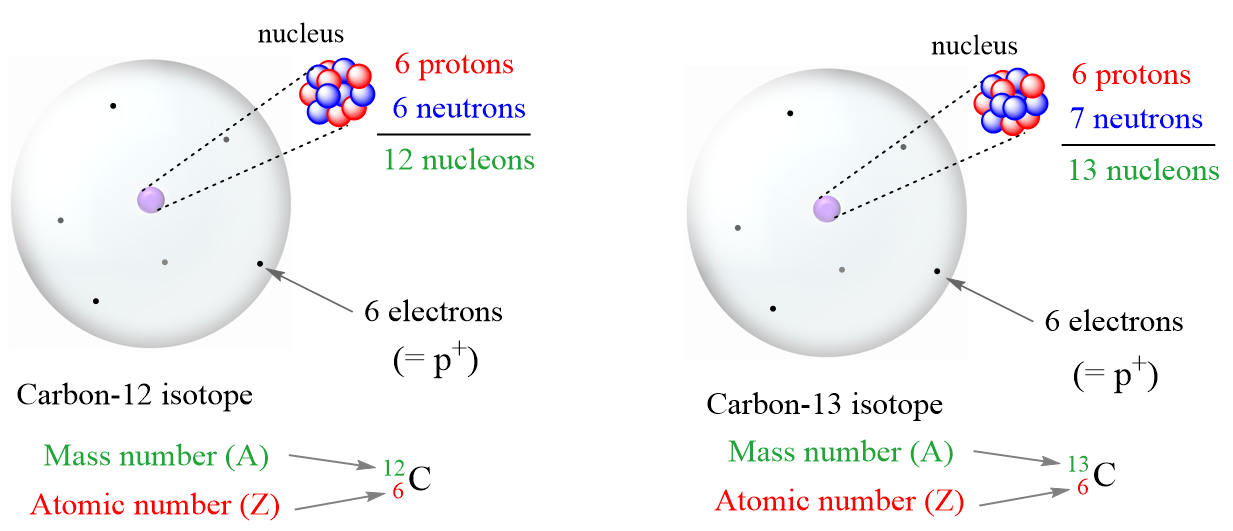# Determining the Number of Protons

In an easy example, you may be asked to simply determine the number of protons for the given element.

For example, how many protons does Ca have?

For this, you just need to look up the atomic number of Ca, which we find, from the periodic table, that it is 20. Therefore, Ca has 20 protons.

Do not get confused if the element is given with some other data such as the atomic mass or the average atomic mass.

For example, how many protons does the following isotope of palladium have?

${}_{46}^{107}{\rm{Pd}}$

Again, we don’t care what isotope it is, what mass, and how many neutrons it has. Pd is always going to have 46 protons as the atomic number is the identity of the element.

## Number of Protons from Neutrons

Suppose you are not given a periodic table, and the questions asks to determine the number of protons from the number of neutrons. In this case, the mass number must be given.

For example, how many protons does the element with a mass number 35 if it has 18 neutrons?

We know that the mass number (A) = number of protons + the number of neutrons, and therefore, the number of protons is equal to:

p = 35 – 18 = 17, and therefore, the element is Cl

## The Number of Protons from Electrons

For a neutral atom, the number of protons and the number of electrons are equal. This is what makes the atom charge-free. Therefore, you can determine the number of protons if the number of electrons is given.

For example, identify the element of an atom with 54 electrons.

All we need to do is look up the element with the atomic number 54, and that is Xe.

# The Number of Protons in Ions

The first thing you need to remember here is the charge of the ion is a result of an imbalance between the number of protons and electrons. If it is a cation, then the positive indicates how many more protons it has compared to the number of electrons. For anions, the charge tells how many extra electrons there are compared to the number of protons.

Recall, this pattern for the formation of anions and cations:

Metals tend to lose electron(s) and become cations (positively charged ions).Nonmetals tend to gain an electron(s) and become anions (negatively charged ions).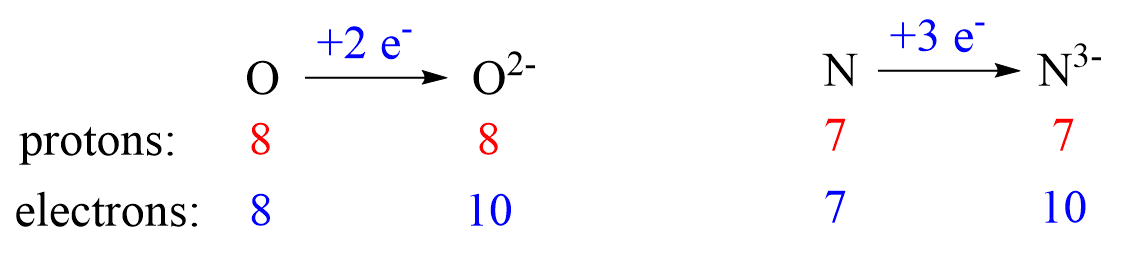Notice that the number of protons is not changed, and the ions are charged because, unlike atoms, their number of protons and electrons are not equal.

For example, what element has a charge of 3+ with 10 electrons?

The 3+ charge indicates that the element has lost 3 electrons. So, if the cation has 10 electrons, the neutral atom must have had 13 electrons because losing 3 of those results in a charge of 3+. And from here, we know that the element has 13 protons because the number of protons is equal to the number of electrons in a neutral atom. The element with atomic number 13 is Al.

# Calculating the Number of Neutrons

The most important part you will need for calculating the number of neutrons is that together with protons, they make the atomic mass of the given isotope. So, if we have the mass number of the given isotope, we can easily determine the number of its neutrons.

For example, how many neutrons does the 65Cu isotope have?

The first thing, again, is to look up the number of protons for Cu, and this is the atomic number. From the periodic table, we find that it has 29 protons. The mass number (65) is the sum of the number of protons and neutrons. Therefore, we can subtract the number of protons from the atomic number to get the number of neutrons:

# n = A – Z = 65 – 29 = 36

## The Number of Neutrons from the Number of Electrons

Because the number of protons and electrons are equal in neutral atoms, we can determine the number of neutrons from the number of electrons and the mass number.

For example, how many neutrons does an atom with a mass of 79 and 35 electrons have?

Having 35 electrons means it also has 35 protons, and if we subtract this from the atomic mass, we will know how many neutrons it has.

# e- = # p = 35

# n = mass number – # p = 79 – 35 = 44

## The Number of Neutrons in Ions

The number of neutrons is always going to be linked to the number of protons and the atomic mass. So, just like we did for the number of protons in an ion, we are going to use the charge to determine the number of neutrons.

For example, how many neutrons are there in an ion with a mass number of 127 and 54 electrons that has a charge of -1?

First, to find the number of protons, we need to realize that the neutral atom had 53 electrons because it is the additional one electron that makes it a 1- anion. Now, because the atom has 53 electrons, it must also have 53 protons, and to find the number of neutrons we subtract this from the mass number.

# n = A – # p = 127 – 53 = 74 neutrons

To summarize, you need to remember these relationships between the atomic mass, the number of protons, neutrons, and electrons:

• Any element has always the same number of protons regardless of what isotope it is.
• The mass number (A) is the sum of the number of protons (atomic number, Z) and neutrons.
• The number of protons and electrons is equal in a neutral atom

More practice examples on average atomic mass and isotopes can be found in this multiple-choice quiz:

#### Practice

1.

Which atom has the smallest number of protons?

a) Li
b) Mn
c) Be
d) Ag
e) As

This content is available to registered users only. Click here to Register! By joining Chemistry Steps, you will gain instant access to the answers and solutions for all the Practice Problems and the powerful set of General Chemistry 1 and 2 Summary Study Guides.
2.

Which atom has the largest number of protons?

a) 11B
b) 14C
c) 14N
d) 19F
e) 18O

This content is available to registered users only. Click here to Register! By joining Chemistry Steps, you will gain instant access to the answers and solutions for all the Practice Problems and the powerful set of General Chemistry 1 and 2 Summary Study Guides.
3.

Which combination of protons, neutrons, and electrons is correct for the 59Ni isotope?

A) 29 p+, 30 n°, 29 e-
B) 28 p+, 30 n°, 28 e-
C) 28 p+, 31 n°, 28 e-
D) 28 p+, 32 n°, 31 e-
E) 27 p+, 32 n°, 27 e-

This content is available to registered users only. Click here to Register! By joining Chemistry Steps, you will gain instant access to the answers and solutions for all the Practice Problems and the powerful set of General Chemistry 1 and 2 Summary Study Guides.
4.

How many electrons are in the O2- ion?

a) 9
b) 10
c) 8
d) 11
e) 6

This content is available to registered users only. Click here to Register! By joining Chemistry Steps, you will gain instant access to the answers and solutions for all the Practice Problems and the powerful set of General Chemistry 1 and 2 Summary Study Guides.
5.

What is the average atomic mass of the element X if it contains 92.23 % of isotope X-27.976, 4.683 % of  X-28.976, and 3.087 % X-29.973?